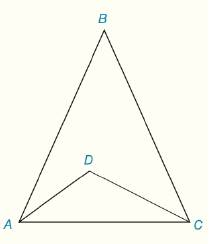Chapter 3.CR, Problem 22CR### Elementary Geometry for College St...

6th Edition
Daniel C. Alexander + 1 other
ISBN: 9781285195698

#### Solutions

Chapter
Section### Elementary Geometry for College St...

6th Edition
Daniel C. Alexander + 1 other
ISBN: 9781285195698
Textbook Problem
1 views

# Given: A B ¯ ≅ B C ¯ ∠ D A C ≅ ∠ B C D m ∠ B = 50 ° Find: m ∠ A D CTo determine

Explanation

Procedure used:

If two sides of the triangle are equal then the corresponding angles are also equal in the triangle.

The sum of interior angles of the triangle is 180°.

Given:

The triangle ΔABC is shown in the figure below,

Figure (1)

From figure (1),

AB¯BC¯DACBCDmB=50°

Calculation:

Consider the triangle ΔABC,

From the given data,

The side AB¯ is congruent to the side BC¯, then the congruent side have same measures. So,

AB¯=BC¯

Since, the two sides of the triangle are equal then the corresponding angles are also equal in the triangle. Then,

mBAC=mBCA

Since, the angle mDAC is congruent to the angle mBCD, then the congruent side have same measures. So,

mDAC=mBCD (1)

Write the equation to calculate the sum of the triangle.

mBAC+mACB+mB=180° (2)

Substitute 50° for mB and mBCA for mBAC in the equation (2) to calculate the sum of the triangle.

### Still sussing out bartleby?

Check out a sample textbook solution.

See a sample solution

#### The Solution to Your Study Problems

Bartleby provides explanations to thousands of textbook problems written by our experts, many with advanced degrees!

Get Started

#### Define sampling with replacement and explain why is it used?

Statistics for The Behavioral Sciences (MindTap Course List)

#### Evaluate the integral. 72. ln(x+1)x2dx

Single Variable Calculus: Early Transcendentals

#### True or False: The slope of a tangent line may be interpreted as average velocity.

Study Guide for Stewart's Single Variable Calculus: Early Transcendentals, 8th